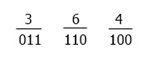# Octal to Binary Conversion

The method that can be used to convert a number from the octal number system to the binary number system has been described in this post, which was created and published in order to provide information about the method. However, before we can understand the method for conversion, it will be beneficial for us to first understand the two different number systems, which are explained in the paragraph that follows this one.

• Octal number: The octal number system has a base of 8, which means this system allows 8 digits to be used to form an octal number. Those 8 digits are 0, 1, 2, 3, 4, 5, 6, 7. For example: 213, 73, and 3023, etc.
Therefore, we can say that a number that consists of 0, 1, 2, 3, 4, 5, 6, and 7 can be considered an octal number.
• Binary number: On the other hand, binary numbers have a base of 2, which indicates that a binary number is comprised of a total of two digits, which are the digits 0 and 1. For example, 11, 11011001, and 1101, etc.

## Octal to Binary Steps

In order to transform any octal number into a binary number, we need to transform each and every octal digit into a binary number that consists of three bits. Take this as an illustration:

Convert the octal number 364 to the binary system. Or (364)8 = ( ? )2.We have three 3-bit binary numbers as a result of the figure that was just presented to us: the first one is 011, the second one is 110, and the third one is 100. As a result, when we combine everything, we get 011110100.

And the result is: (364)8 = (011110100)2.

## Octal to Binary Conversion Table

With the help of the following table, any octal number can be converted into a binary number: It is necessary to convert each octal digit into its corresponding binary value, which must be done one at a time. The following table presents, in binary notation, the equivalent of each of the eight octal digits:

Octal Digit Equivalent Binary Value
0 000
1 001
2 010
3 011
4 100
5 101
6 110
7 111

## Octal to Binary Example

For example, let's suppose the number 254 is given as an octal number; therefore, to convert it into binary, you have to write the binary equivalent of each octal digit one by one. The octal digits are 2, 5, and 4. Therefore, the binary equivalents of 2, 5, and 4 octal digits are 010,  101, and 100, respectively. That is, (254)8 = (010101100)2.

#### Programs Created on Octal to Binary Conversion

Computer Fundamentals Quiz

« Previous Tutorial Next Tutorial »